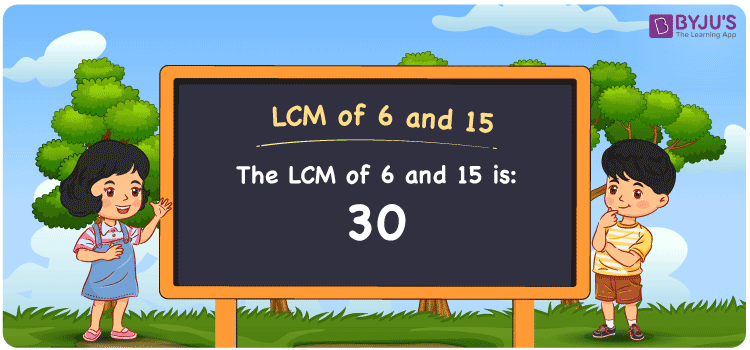Checkout JEE MAINS 2022 Question Paper Analysis : Checkout JEE MAINS 2022 Question Paper Analysis :

# LCM of 6 and 15

LCM of 6 and 15 is 30. The smallest number which is evenly divisible among the given multiples of the two numbers indicates the LCM. By understanding the common multiples, the least common multiple of 6 and 15 can be obtained. (6, 12, 18, 24, 30, 36, 42, ….) and (15, 30, 45, 60, 75,….) are the multiples of 6 and 15. The LCM can be calculated effectively using a few methods like prime factorisation, division method and by listing out the multiples.

## What is LCM of 6 and 15?

The answer to this question is 30. The LCM of 6 and 15 using various methods is shown in this article for your reference. The LCM of two non-zero integers, 6 and 15, is the smallest positive integer 30 which is divisible by both 6 and 15 with no remainder.## How to Find LCM of 6 and 15?

LCM of 6 and 15 can be found using three methods:

• Prime Factorisation
• Division method
• Listing the multiples

### LCM of 6 and 15 Using Prime Factorisation Method

The prime factorisation of 6 and 15, respectively, is given by:

6 = 2 x 3 = 2¹ x 3¹

15 = 3 x 5 = 3¹ x 5¹

LCM (6, 15) = 30

### LCM of 6 and 15 Using Division Method

We’ll divide the numbers (6, 15) by their prime factors to get the LCM of 6 and 15 using the division method (preferably common). The LCM of 6 and 15 is calculated by multiplying these divisors.

 2 6 15 3 3 15 5 1 5 x 1 1

No further division can be done.

Hence, LCM (6, 15) = 30

### LCM of 6 and 15 Using Listing the Multiples

To calculate the LCM of 6 and 15 by listing out the common multiples, list the multiples as shown below

 Multiples of 6 Multiples of 15 6 15 12 30 18 45 24 60 30 75 36 90

LCM (6, 15) = 30

## Video Lesson on Applications of LCM## LCM of 6 and 15 Solved Examples

If the product of two numbers is 90 and the GCD is 3, find the LCM.

Solution:

It is given that;

Product of two numbers = 90

GCD = 3

We know that

LCM x GCD = Product of two numbers

LCM = Product/GCD

LCM = 90/3

LCM = 30

Hence, the LCM is 30.

## Frequently Asked Questions on LCM of 6 and 15

### Determine the LCM of 6 and 15.

The LCM of 6 and 15 is 30.

The smallest multiple which is exactly divisible by 6 and 15 has to be found.

Multiples of 6 = 6, 12, 18, 24, 30, 36, …

Multiples of 15 = 15, 30, 45, 60, 75, ….

The smallest common multiple exactly divisible by 6 and 15 is 30.

### What are the methods used to find the LCM of 6 and 15?

The methods used to find the LCM of 6 and 15 are the Prime Factorization Method, Division Method and Listing multiples.

### Find the GCF if the LCM of 6 and 15 is 30.

LCM x GCF = 6 x 15

Given

LCM of 6 and 15 = 30

30 x GCF = 90

GCF = 90/30 = 3